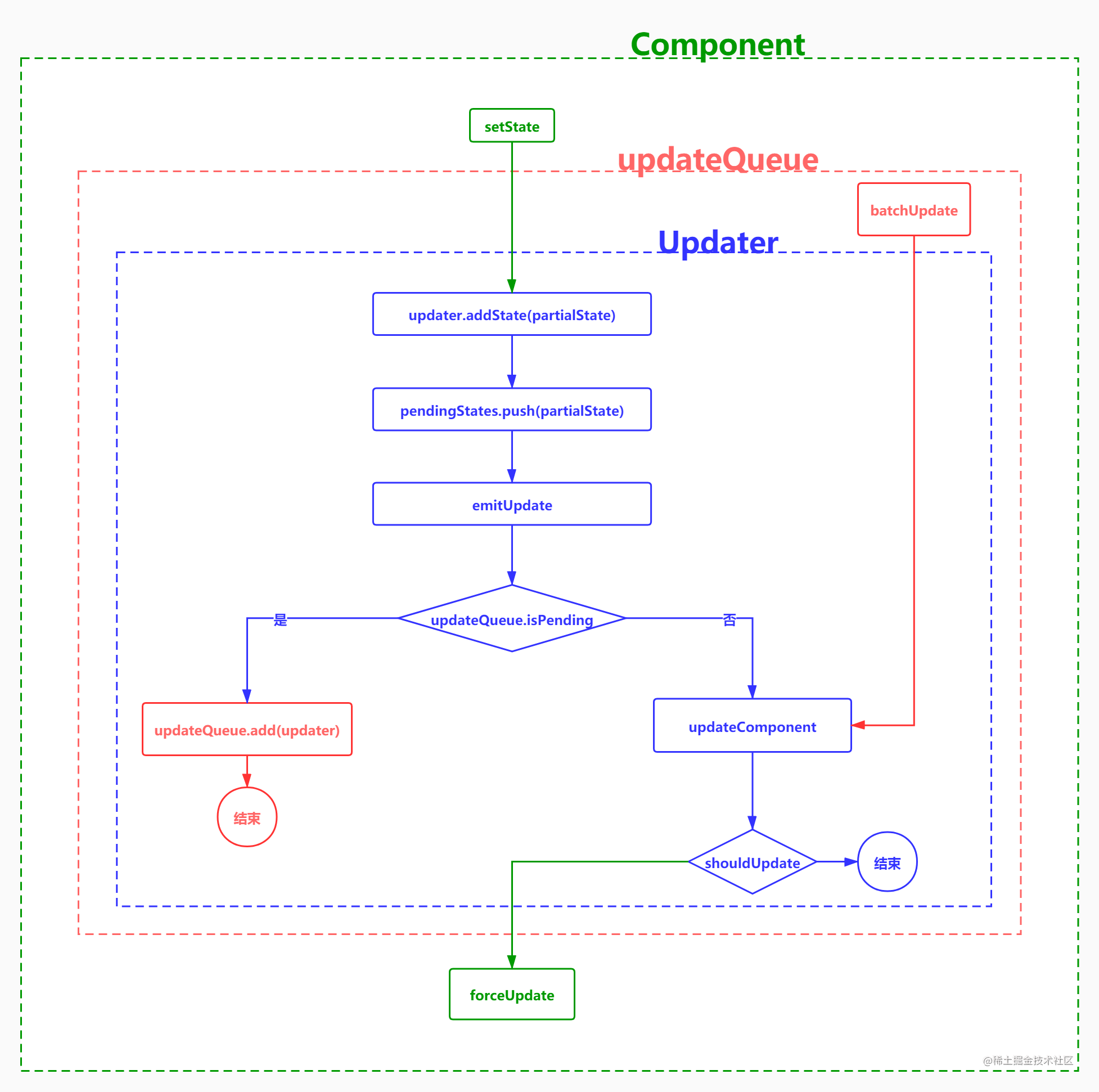# 你知道 React setState 的原理吗？

## 现象描述

``````import React from 'react'

export class SetStateDemo extends React.Component {
constructor(props) {
super(props)
this.state = { number: 0 }
}

render() {
return (
<div>
<p>{this.state.number}</p>
<button onClick={this.handleClick}>+</button>
</div>
)
}

handleClick = () => {
this.setState({ number: this.state.number + 1 })
}
}

``````  handleClick = () => {
this.setState({ number: this.state.number + 1 })
this.setState({ number: this.state.number + 1 })
}

``````  handleClick = () => {
setTimeout(() => {
this.setState({ number: this.state.number + 1 })
this.setState({ number: this.state.number + 1 })
})
}

## 原理分析

``````  handleClick = () => {
this.setState({ number: 1 })
this.setState({ number: 2 })
this.setState({ number: 3 })
this.setState({ number: 4 })
this.setState({ number: 5 })
}### 非批量更新

``````import { createDOM } from './react-dom'

class Component {
static isReactComponent = true
constructor(props) {
this.props = props
this.state = {}
}
// 非批量更新的 setState 实现
setState(nextState, callback) {
if (typeof nextState === 'function') nextState = nextState(this.state)
this.state = { ...this.state, ...nextState }
this.forceUpdate()
if (typeof callback === 'function') callback()
}
​
// 强制刷新
forceUpdate() {
this.componentWillUpdate?.() // 调用 componentWillUpdate 钩子
const oldDOM = this.dom // 类组件当前对应的真实DOM
const newDOM = createDOM(this.render()) // 新的DOM
oldDOM.parentNode.replaceChild(newDOM, oldDOM) // 替换
this.dom = newDOM
this.componentDidUpdate?.() // 调用 componentDidUpdate 钩子
}
​
render() {
throw new Error('此方法为抽象方法，需要子类实现')
}
}

### 批量更新

``````export const updateQueue = {
isBatchingUpdate: false, // 是否处于批量更新模式
updaters: new Set(),
batchUpdate() {
for (let updater of updateQueue.updaters) {
updater.updateClassComponent()
}
updateQueue.isBatchingUpdate = false
},
}

// 专门负责更新的类
class Updater {
constructor(it) {
this.classInstance = it // 类组件的实例
this.pendingStates = [] // 等待生效的状态，可能是一个对象，也可能是一个函数
this.callbacks = [] // 回调数组
}

this.pendingStates.push(partialState) // 缓存用户设置的新状态（可能是函数或对象）
if (typeof callback === 'function') this.callbacks.push(callback)
this.emitUpdate() // 通知更新
}

// 无论属性变了还是状态变了，都会更新
emitUpdate() {
if (updateQueue.isBatchingUpdate) {
} else {
this.updateClassComponent() // 更新函数组件
}
}

// 立即更新函数组件
updateClassComponent() {
const { classInstance, pendingStates, callbacks } = this
if (pendingStates.length > 0) {
const nextState = this.getState() // 计算新状态
classInstance.state = nextState

/* 生命周期钩子拒绝更新的场景 */
const flag = classInstance.shouldComponentUpdate?.(classInstance.props, nextState)
if (flag === false) return

classInstance.forceUpdate() // 强制更新
callbacks.forEach(cb => cb())
callbacks.length = 0
}
}

// 获取批量更新最后的状态
getState() {
let { state } = this.classInstance
this.pendingStates.forEach(nextState => {
if (typeof nextState === 'function') nextState = nextState(state)
state = { ...state, ...nextState }
})
this.pendingStates.length = 0
return state
}
}

### 合成事件

``````handleClick = () => {
updateQueue.isBatchingUpdate = true
this.setState({ number: this.state.number + 1 })
this.setState({ number: this.state.number + 1 })
updateQueue.batchUpdate()
}

``````handleClick = () => {
updateQueue.isBatchingUpdate = true
setTimeout(() => {
this.setState({ number: this.state.number + 1 })
this.setState({ number: this.state.number + 1 })
})
updateQueue.batchUpdate()
}

• 可以对所有事件处理函数内置批量更新逻辑
• 可以屏蔽不同浏览器对 DOM 事件实现的差异